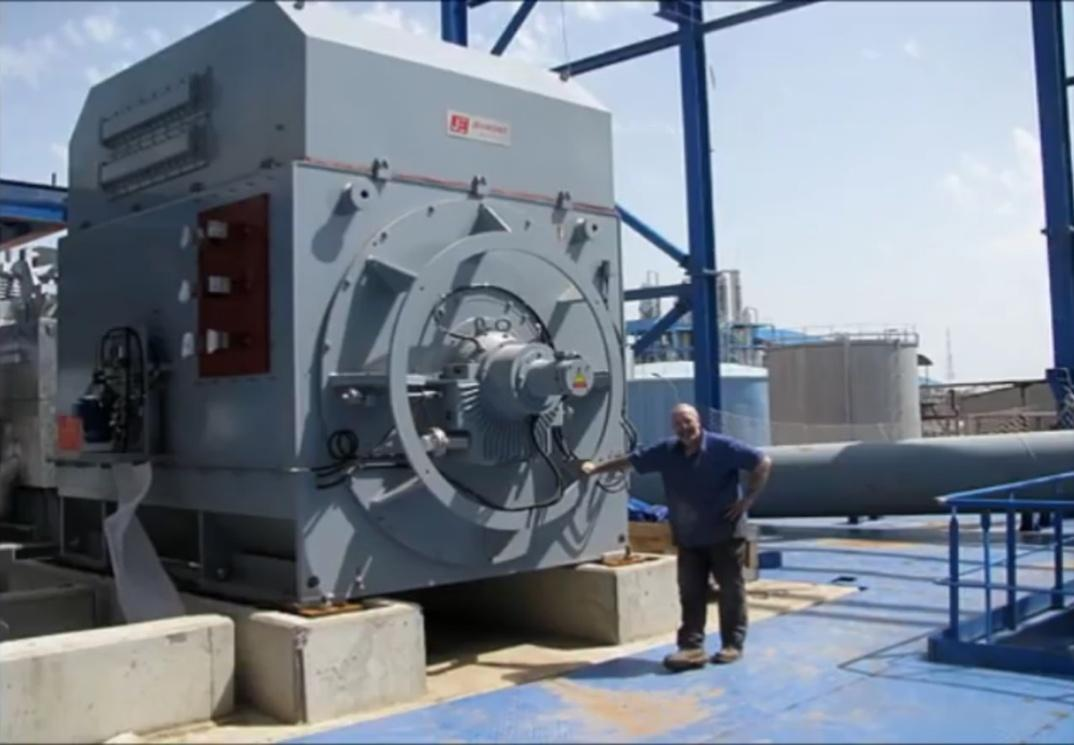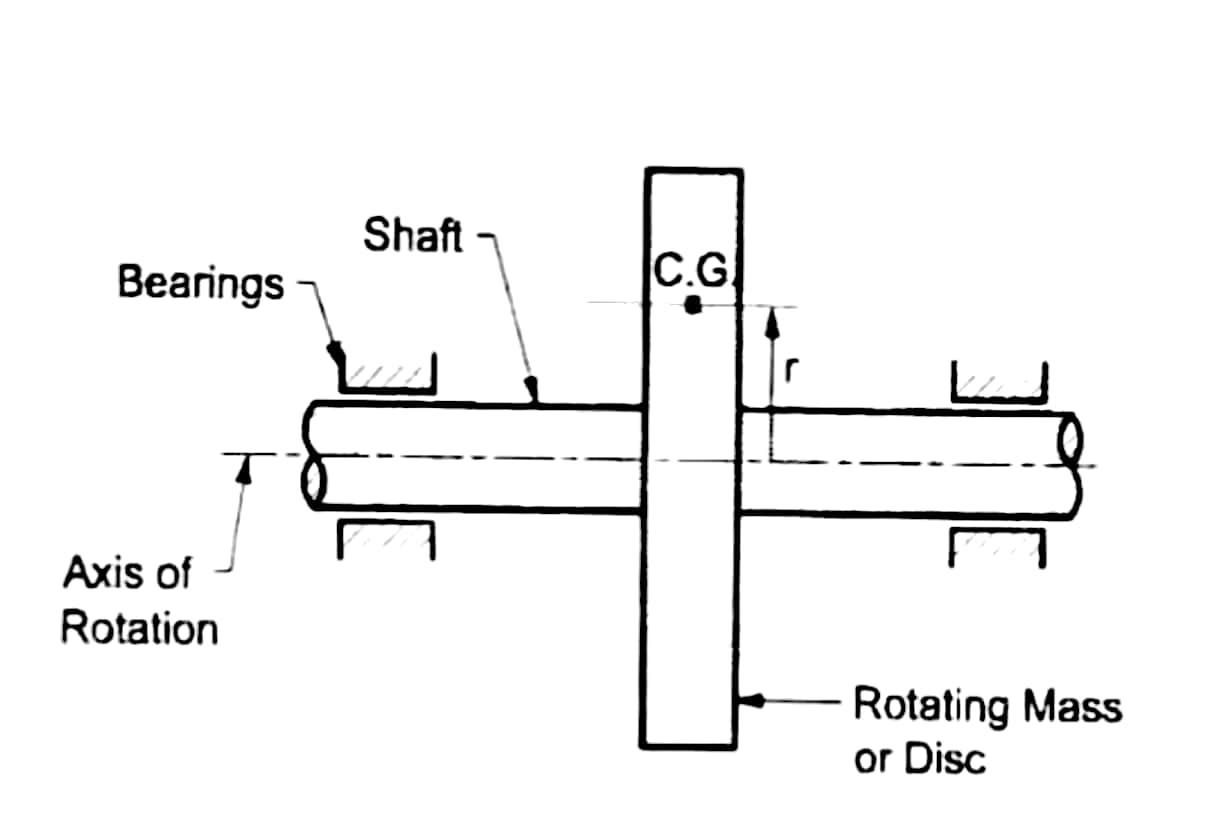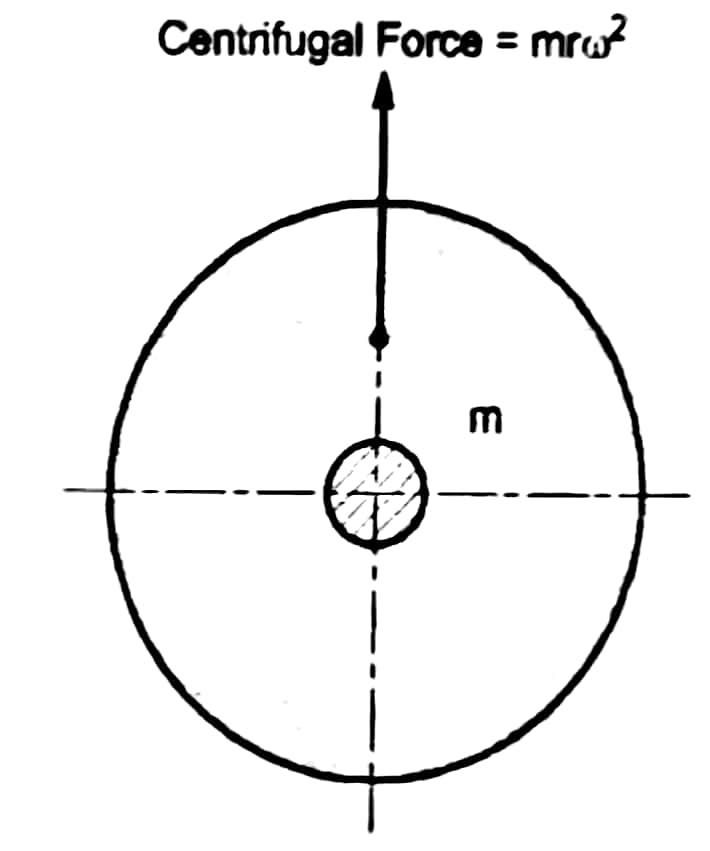## Static and Dynamic Balancing

When machine is in working condition different forces are acting on it which may cause machine to vibrate and cause damage to machine parts. The different forces acting on machine parts are static forces and dynamic forces.

### Static force

The force which depends on weight of a body, is known as static force. (Generally, a static force acts when vibrations occurs in same plane.)

### Dynamic or Inertia force

The force which depends on acceleration of a body, is known as dynamic force. (Generally, dynamic force acts when vibrations occurs in different planes.)

Due to these forces, the efficiency of the system decreases and life span of the system also decreases.
Due to these forces, the machine starts vibrating and sometimes when the vibrations increases, the machine would lift from it’s position and cause damage to other machine or human. So to avoid this, foundation is made below the machine (as you can see in the Fig-1 below), which absorbs the vibration and protects the machine against causing damage. Thus, balancing of the machine is required.Fig-1

### Balancing

Balancing is the process of eliminating, the effect of static forces and dynamic forces acting on machine components.

## When is system said to be unbalanced?

In any system with one or more rotating masses, if the centre of mass of the system does not lie on the axis of rotation, then the system is said to be unbalanced.

As you can see in the Fig-2 we have a rotor which is mounted on a shaft and the shaft has its own axis of rotation. Now you can see that the C.G(centre of gravity) of the rotor is at a distance r from the axis of rotation of the shaft, so when the rotor will start to rotate, a centrifugal force will act on it which is in the outward direction as you can see in Fig-3. Due to this force our system will become unbalanced and it will start to vibrate.Fig-2Fig-3

### Static balancing

A system is said to be statically balanced, if the centre of masses(C.G) of the system lies on the axis of rotation.

#### Condition for Static Balancing

The resultant of all the centrifugal forces (dynamic forces) acting on the system during rotation must be zero.

\begin{aligned} \small ∑ \text {Centrifugal forces acting on the system} &= \text {zero}\\ i.e. \ +mrω^2 – mrω^2 &= 0 \end{aligned}

### Dynamic balancing

A system is said to be dynamically balanced, if it satisfies following two conditions:-

1. The resultant of all the dynamic forces acting on the system during rotation must be zero.
$∑ \text {Dynamic forces acting on the system} = \text {zero}$
1. The resultant couple due to all the dynamic forces acting on the system during rotation, about any plane, must be zero.
$∑ \text {couple} = \text {zero}$